Home > CC4 > Chapter 11 > Lesson 11.1.1 > Problem11-10

11-10.
1. Solve the following inequalities for the given variable and represent the solutions on a number line. 11-10 HW eTool (CPM). Homework Help ✎

2.  a. 2 < 2m – 8 b.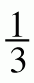x – 1 < –3 c. 5(2x – 8) + 24 > 3(4 + 2x) d. 5 + 2k < k – 2 + k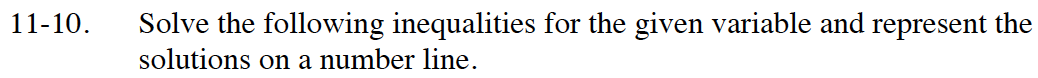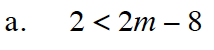Solve as an equation and test a point to decide where to shade.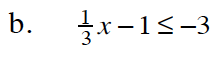Remember to multiply by a common denominator to remove the fraction.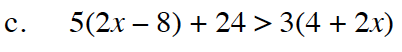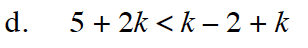Use the eTool below to represent the solutions on number lines.
Click the link at right for the full version of the eTool: Int1 11-10 HW eTool